# Civil Engineering - Water Resources Engineering

### Exercise :: Water Resources Engineering - Section 2

36.

If the grain size of soil increases

 A. surface area decreases B. specific retention decreases C. specific yield increases D. water supply in well increases E. all the above.

Explanation:

No answer description available for this question. Let us discuss.

37.

The equation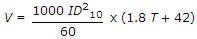which is used for determining the velocity of ground water flow in metres per day is known as

 A. Meinzer's formula B. Slichter's formula C. Darcy's formula D. Hazen formula.

Explanation:

No answer description available for this question. Let us discuss.

38.

Pick up the correct statement from the following :

 A. A confined bed of impervious material laid over an acquifer, is known as an aquiclude B. The top most water bearing strata having no acquifer, is known as non-artesion acquifer C. The ordinary gravity wells which supply water from the top most water bearing strata, are called water table wells D. A permeable stratum which is capable to yield appreciate quantities of ground water, is known as an aquifer E. All the above.

Explanation:

No answer description available for this question. Let us discuss.

39.

While determining the yield of a gravity well by pumping, the depth of water table in two tests wells at distances r1 and r2 from the centre of the main well were found to be s1 and s2 respectively. Assuming the coefficient of transmissibility of the soil as T, the discharge Q may be given by

 A.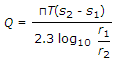B.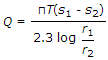C.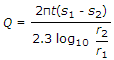D.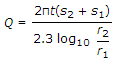Explanation:

No answer description available for this question. Let us discuss.

40.

According to Thiem, the permeability of an aquifer may be obtained from the equation

 A.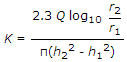B.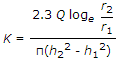C.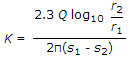D.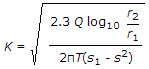E. all the above.